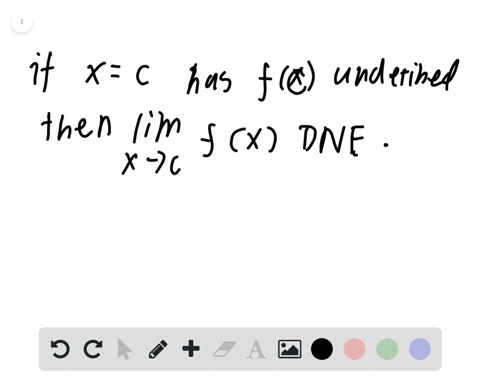Enroll in one of our FREE online STEM bootcamps. Join today and start acing your classes!View Bootcamps### True or False? In Exercises $73-76,$ determine wh…

00:44
FS
University of California, Berkeley
Problem 72

# HOW DO YOU SEE IT? Use the graph of $f$ to identify the values of $c$ for which lim $f(x)$ exists.

## Discussion

You must be signed in to discuss.

## Video Transcript

Okay, so this is a two part question here. Were given a graph off at prime and where has to approximate a slope of at and actually negative one now, because the slope is the driven, the function f every different point. We have to find ash prime of negative. Born using the graph of a crime. So we have to do is look up negative one. And it gets to you that this is approximately negative. One over two Part B asks us approximate any open intervals on which the graph of F is increasing and any organ it trickles and wished a graph of is decreasing. So we know that is F Crime of X is greater than zero for all acts. Then app is increasing so this implies that is increasing and similar. The crime of axe is less than zero. It implies that ass is decreasing on those intervals. So, as you can see on the graph after X is greater than zero for all acts in the range of negative too to infinity. And it is less than zero for all access in the range of native infinity, two native to all right. That's all

#### You're viewing a similar answer. To request the exact answer, fill out the form below:

Our educator team will work on creating an answer for you in the next 6 hours.